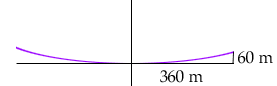Subject: applications of quadratic relations Janna Davies student secondary question I found the equation of a parabola for a problem, but then tried to substitute a number in and it didn't work! Here's the whole question: The cables of a suspension bridge hang in a curve which approximates a parabola. The road bed passed through the vertex. If the supporting towers are 720m apart and 60m high, find: a) an equation of the parabola (it's y = 1/2160x2) b)the height of the cables at a point 30m from the vertex. I substituted 30 in for the x value and got 0.42 and the answer is 42. What did I do wrong? Hi Janna, Below is a diagram that is approximately to scale.When you are on the x-axis, 30 m from the vertex of the parabola, the height of the curve can't be anywhere near 42 m high. Your answer of 0.42 m is exactly what we get. The height of 42 m comes at 300 m from the vertex. Cheers, Denis and Claude Go to Math Central